Subscribe to our newsletter to receive the latest news and events from TWI:

# Limit Load and Reference Stress for Pipe Girth Weld Flaws

## Approaches for Determining Limit Load and Reference Stress for Circumferential Embedded Flaws in Pipe Girth Welds

TWI Ltd Granta Park, Great Abington, Cambridge CB21 6AL, UK

Pipeline Technology Conference, Ostend, Belgium 2009.

### Abstract

This paper provides an evaluation of approaches for determining limit load (and equivalent reference stress) for use in FAD-based fracture assessment of circumferential embedded flaws in pipe girth welds. Three-dimensional elastic plastic finite element analyses have been conducted on pipe models containing typical circumferential embedded flaws and subject to global bending load. ‘J-based’ limit loads (and equivalent reference stress) and global collapse limit loads have been determined from the finite element analyses and used to evaluate existing standard flat plate solutions, including those in BS 7910 and R6. A general approach for determining limit load (and equivalent reference stress) is presented. This approach is consistent with both the finite element results and reference stress J estimation scheme and, consequently, allows the development of improved assessment models.

### Introduction

The use of an engineering critical assessment (ECA) to derive flaw acceptance criteria for pipeline girth welds allows the maximum tolerable size of surface and embedded circumferential planar flaws to be determined on a fitness-for-purpose basis. A typical ECA involves assessing the significance of such flaws with regard to failure mechanisms, including fracture, which the pipeline may experience during construction, commissioning and service. The most commonly used approach to assess the significance of flaws with regard to fracture and plastic collapse is the failure assessment diagram (FAD), which is based on the reference stress J estimation scheme (BSI, 2005) and (BEGL, 2001). A FAD-based assessment involves the calculation of a fracture parameter (Kr,√δr or Jr) and a plastic collapse parameter, Lr, Figure 1. The fracture parameter characterises the proximity to fracture under linear elastic conditions. The plastic collapse parameter characterises the proximity to failure by yielding mechanisms and is defined as either the ratio of applied load to the limit load or, equivalently, the ratio of the reference stress to the yield stress. There is a unique relationship between a reference stress and a limit load, which enables one to be defined if the other is known. Specifically, a limit load is inversely proportional to the corresponding reference stress as follows: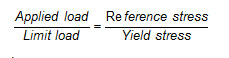(1)

The work described in this paper concerns the development of a reference stress (or limit load) model for use in FAD-based fracture assessments of circumferential embedded girth weld flaws such as that shown in Figure 2. This work is considered necessary since existing reference stress solutions for embedded flaws are not consistent with reference stress solutions that are typically used to assess surface flaws because the approaches used in their derivation are different. Whereas there are several reference stress models for surface breaking flaws, including some intended specifically for circumferential flaws in pipe sections, there are fewer reference stress models for embedded flaws and these are intended for flaws in flat plates.

One of the most widely used solutions is that in BS 7910:2005 (BSI, 2005), which assumes that plastic collapse occurs locally when the net section stress on a small area surrounding the embedded flaw reaches the yield or flow strength of the material. It also assumes that tensile loads are applied through pin-jointed coupling, ie that there is negligible bending restraint. The use of this solution to assess embedded flaws could lead to overly conservative results, which may in some cases be counter-intuitive, eg for a given applied loading, the maximum tolerable length for an embedded flaw (whose ligament is greater than or equal to the height of one weld pass) is smaller than that for a surface breaking flaw of the same height.

The paper focuses on the evaluation of existing reference stress (and equivalent limit load) solutions for embedded flaws and the development of improved models. Existing solutions are evaluated using data generated from elastic-plastic finite element analyses of pipe models containing typical circumferential embedded flaws.

### Scope of Work

The scope of work includes three-dimensional elastic-plastic finite element analyses of pipe models containing typical circumferential embedded flaws. The pipe models are loaded by pure bending, which is the pre-dominant loading mode during pipeline installation. The models are perfectly aligned across the section containing the flaw and the same tensile properties are assigned to the parent and weld metals. Data from the finite element analyses are used to review and evaluate a number of methods for determination of the reference stress (or limit load) for embedded flaws including conventional methods, recommended in BS 7910 (BSI, 2005) and R6 (BEGL, 2001), and novel methods. It is shown that none of the existing codified reference stress (or limit load) solutions agree well with results obtained from the finite element analyses. Therefore, a new approach using a novel method for definition of reference stress, which is fully consistent with findings from the finite element analyses, is proposed.

The remainder of this paper consists of the following sections: reference stress J estimation scheme; approaches for determining reference stresses (and limit loads) in FAD-based assessments; codified reference stress (and limit load) solutions for embedded flaws; approach adopted for determining J-based limit loads (MJ); scope of (and results from) finite element analyses; comments on global collapse and J-based limit loads; comparison of flat plate solutions with J-based solutions; plastic strain in ligament; summary and conclusions.

### Reference Stress J Estimation Scheme

The evaluation of reference stress models is performed within the context of the reference stress J estimation scheme (BEGL, 2001), which is defined below.

J is estimated from the following expressions, which correspond to the material-specific FAD (BS 7910 Level 2B/3B and R6 Option 2):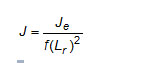(2)

and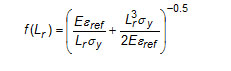(3)

where for a given applied bending moment, M, Lr σy is the reference stress (denoted as σref); εref is the reference strain corresponding to sref and determined from the stress-strain curve of the material; σy is the yield or 0.2% proof strength of the material and E is Young’s modulus. Je is the elastic value of J, at an applied moment M, determined from data obtained at an applied moment Mo, as follows: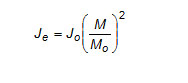(4)

or using the equivalent expression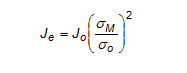(5)

where, Jo is the elastic value of J determined at Mo (eg from an elastic finite element analysis), σM and σo are the elastic bending stresses on the pipe OD corresponding to M and Mo, respectively, determined using elastic section properties.

An alternative estimate of the elastic value of J may be determined as follows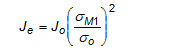(6)

which is similar to Equation  but uses the actual elastic-plastic stress on the pipe OD (denoted σM1), rather than σM. In this case, Je is not proportional to M2.

The parameter Lr, which characterises the proximity to plastic collapse, can be expressed as follows: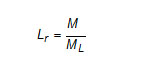(7)

or alternatively as: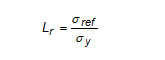(8)

where ML is the limit load.

The reference stress J-estimation scheme could also be applied using alternative expressions of J, eg which correspond to simplified FADs in BS 7910 and R6.

There are a number of approaches for determining ML (and the corresponding σ ref ), which are described in the following sections

The limit load (or plastic collapse) required for the calculation of reference stress and the parameter Lr is one of the most important elements of a FAD-based assessment since it serves two functions:

• It ensures that the limit load of the component containing the flaw under consideration is not exceeded.
• It ensures that the relationship between elastic-plastic driving force and proximity to plastic collapse is consistent with the relationship implied by the failure assessment curve.

Two potential plastic collapse modes of a flawed component can be identified:

• Local collapse: corresponds to failure, by yielding mechanisms, of the ligament adjacent to the flaw. This is deemed to occur when the stress in the remaining ligament reaches the yield strength of the material. With regard to a circumferential part-thickness flaw in a girth weld (surface breaking or embedded), the significance of the circumferential extent of the remaining ligament on either side of the flaw is not well defined in any of the existing standards. Another source of uncertainty is whether bending of the section containing the flaw is restrained or not. In the absence of bending restraint, secondary bending stresses arise due to eccentric loading. This is caused by movement of the neutral axis, due to the existence of the flaw, compared with the unflawed condition.
• Global collapse: corresponds to failure, by yielding mechanisms, of the whole section containing the flaw. This is deemed to occur when the global deformation, displacement and/or rotation, of the section become unbounded. Global collapse occurs at a higher load than that corresponding to local collapse.

Most codified limit load or reference stress solutions for part-thickness (surface or embedded) flaws are based on the local collapse approach. Although some standards, such as R6 (BEGL, 2001) provide also solutions based on the global collapse approach further checks, eg against finite element analyses, may be required to verify that such solutions provide safe assessments.

An alternative method for determining limit loads, which requires J data from finite element analyses, consists of defining the limit load such that it is consistent with the J data and reference stress J estimation scheme represented by Equation . The main benefit of this approach is that it does not require the analyst to specify in advance whether a local or global collapse model is more suitable. The limit load is found by solving Equations  and  using J results from an elastic-plastic finite element analysis of the flawed component. A simple version of this approach is recommended in Section B.6.4.3(e) of API 579 (API, 2000) and in a slightly different form in Section B.1.89 of API-579-1/ASME-FFS-1 (API/ASME, 2007) as follows: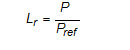(9)

where Pref is determined from the following relationship: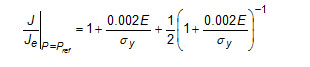(10)

where J is the total value of J determined from an elastic-plastic analysis of the flawed component; Je is the elastic J determined from an elastic analysis, eg using Equations  or ; P is a characteristic applied load (or stress) such as axial force, bending moment or a combination thereof; and Pref is the reference load (or stress) defined as the load at which the ratio J/Je reaches the value defined by Equation .

If Pref is used to construct a BS 7910 Level 3C FAD (with Lr defined according to Equation ), it will intersect the corresponding BS 7910 Level 2B/3B material-specific FAD, ie give the same Kr value, at Lr = 1. Thus, the limit load Pref is defined in a manner, which is consistent with the Level 2B/3B material-specific FAD at least at Lr = 1.0. In this case, the limit load may depend on the strain-hardening characteristics of the material.

### General

Whereas there are several well-established reference stress solutions for circumferential surface flaws in pipe girth welds, there are no such solutions for circumferential embedded flaws. Consequently, most analysts use reference stress solutions originally derived for flat plates to assess circumferential embedded flaws in pipe girth welds. The most widely used of these solutions are the reference stress equations given in BS 7910 (BSI, 2005) and R6 (BEGL, 2001). These are given in terms of the membrane stress, Pm, and through wall bending stress, Pb. However, given that in a thin-walled pipe loaded by bending, Pb is very small compared with Pm, and in a pipe loaded by tension, Pb is equal to zero, the reference stress equations considered below are given assuming that Pb is equal to zero. Note that references to equations, sections and figures in codes and standards are shown in italic.

### BS 7910

The embedded flaw reference stress equation in Equation P4 of BS 7910 (BSI, 2005) is based on local collapse and assumes that tensile loads are applied through pin-jointed coupling, ie that there is negligible bending restraint. The equation was derived by Willoughby and Davey (1987) assuming that the load-bearing area (or ligament) extends to the plate surfaces above and below the embedded flaw and has a length equal to the flaw length plus one plate thickness on either end of the flaw. The solution, with Pb set at zero, is as follows: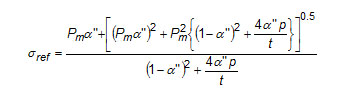(11)

where p is the ligament (smallest distance between the flaw and surface), t is the wall thickness, and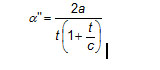(12)

where 2a is the flaw height and 2c is its length, see Figure 3 (note that the wall thickness in BS 7910 is denoted as B).

### R6

R6 (BEGL, 2001) provides several reference stress solutions for embedded flaws in flat plates, which are based on local or global collapse with loads applied through pin-jointed coupling (ie pin loading) or fixed-grip loading conditions. The approach used to develop these solutions is described by Lei and Budden (2004). The solutions cater for flaws located fully or partially in the tensile stress zone (determined by the location of the neutral axis). Whilst this distinction is important when assessing flat plates, it is less significant for circumferential embedded flaws in pipe girth welds, which are almost always assumed to be in the tensile stress zone. Consequently, attention is focussed below on the latter condition. The solutions are expressed using the following parameters: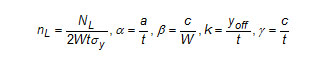where NL is the limit load and other parameters are illustrated in Figure 4. The limit load solutions are given below in terms of the non-dimensional parameter nL.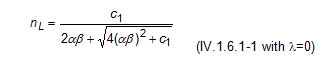(13)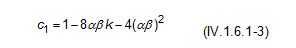(14)

Valid for: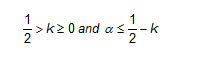Global collapse, fixed-grip tension (IV.1.6.3-1 with k=0):

As above (Equations  and ) but with k = 0 (limit load does not depend on crack position in the cross section).

Local collapse, pin-loaded (IV.1.6.3.2), solution (a):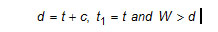This solution is approached when yielding takes place across the whole loading-bearing area 2(t + c) t.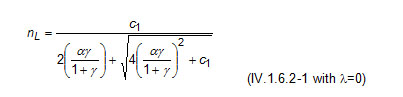(15)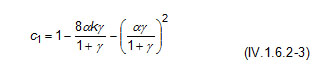(16)

Valid for: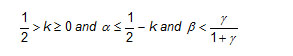Local collapse, pin-loaded (IV.1.6.3.2), solution (b):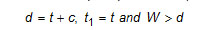This is approached when yielding spreads through the smallest ligament along the plate thickness. Solution (b) is always less than or equal to Solution (a).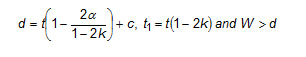(17)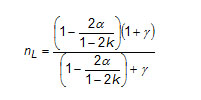(18)

Local collapse, fixed-grip tension: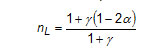(19)

As evident from the above, there are numerous approaches for the definition of limit load including local collapse, global collapse, or J-based methods associated with the reference stress J estimation scheme. Each of these approaches can be applied using a number of options. For example, local collapse can be defined based on a somewhat arbitrarily postulated load-bearing area, and either fixed-ended or pin-ended supports (reflecting whether bending of the section containing the flaw is restrained or not). In practice it is difficult to assess the suitability of these solutions for use in specific applications without additional information.

• Lr is determined by solving Equations  and .
• MJ is determined as M/Lr (according to Equation ), where M is the applied moment.

This approach allows MJ to be determined for every load increment and, consequently, allows MJ to be plotted as a function of any loading parameter, including applied moment, remote applied stress/strain or Lr.

In theory, MJ may depend on the position along the crack front. In the present work, attention has been restricted to solutions in the centre of the crack, which is usually the position of maximum J.

## Finite Element Analyses

Three-dimensional elastic-plastic finite element analyses were conducted on pipe models containing a range of embedded flaw geometries. Four pipe geometries with pipe radius to thickness ratios in the range 5 to 20 were considered. In this paper, only results from Series E1 (pipe outside diameter =400mm, wall thickness = 20mm), which were conducted using the stress-strain curve shown in Figures 5a and b, are reported. The stress-strain curve was constructed from the following Ramberg-Osgood power-law hardening relationship: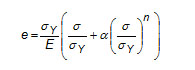(20)

where:

e = true strain.

σ = true stress.

σY = 0.2% proof strength (= 400MPa).

E = Young’s modulus (200,000MPa).

n = strain hardening exponent (= 15).

α = 0.002/eY (= 1.0).

eY = σY/E.

Each finite element model contained an elliptical embedded flaw oriented in the circumferential direction and contained within a plane perpendicular to the pipe axis. The flaws considered had a height in the range 3 to 9mm, were located at 1.5 to 14mm from the pipe inside surface and had a length, along the ellipse major axis, in the range 25 to 250mm. The flaws were located at the 12 o’clock position to ensure that they were subjected to the largest tensile stresses when the model was loaded by bending. The models were loaded by pure bending, which is the predominant loading mode during pipeline installation. In all models, the pipes were perfectly aligned across the section containing the flaw and the same tensile properties were assigned to the finite elements representing parent and weld metals. The dimensions of the pipes and flaws considered within Series E1 are summarised in Table 1.

All analyses were performed using ABAQUS Version 6.6 using a small strain formulation, which is considered to be acceptable at least up to applied strains in the range 0.5 to 1%. The finite element meshes consist entirely of type C3D20R elements, which are 20-noded, quadratic, three-dimensional elements. As the flaw in the pipe is symmetric about the vertical plane and the applied load is also symmetric about this plane, it is only necessary to model half the pipe.

In addition to analyses using the abovementioned stress-strain model, analyses were performed on a number of pipe models using an elastic-perfectly plastic stress-strain model to determine a global collapse limit load, denoted MFEA.

## Results

Analyses using the above stress-strain model enabled the J-integral to be calculated as a function of the applied load along the crack front. The highest J values, which generally occurred at the middle of the crack front adjacent to the smaller of the two ligaments (below and above the flaw), were adopted.

The J results were used to estimate the following J-based limit loads according to the approach described earlier:

• MJ2B E (consistent with Equation , representing the material-specific FAD of BS 7910 (Level 2B/3B) and R6 (Option 2) with Je estimated using the elastic pipe bending stress according to Equation ).
• MJ2B EP (consistent with Equation , representing the material-specific FAD of BS 7910 (Level 2B/3B) and R6 (Option 2) with Je estimated using the elastic-plastic pipe bending stress according to Equation ).

The above results are shown in Figures 6 and 7 in terms of MJ2B EP /4tσyRm 2 vs. Lr and applied strain, respectively. Here, t is the pipe thickness, Rm is the pipe mean radius, and sy is the pipe yield strength. Plots of MJ2B E /4tsyRm 2 vs. Lr and applied strain are not included since they have broadly similar shapes to those shown in Figures 6 and 7 (but MJ2B E is approximately 4% higher than MJ2B EP).

Figures 6 and 7 indicate that MJ2B EP increase slightly with Lr (for Lr > 1.0) and applied strain (for strains > 0.2%). This behaviour is believed to be due to a number of factors including:

• The fact that J-based limit loads are not true limit loads: MJ2B E is lower than MFEA, which is a true global collapse limit load, by approximately 9 to 16%; MJ2B EP is lower than MFEA by approximately 13 to 19%.
• Approximations within the reference stress J estimation scheme.

The fact that MJ (ie MJ2B E and MJ2B EP) increases with Lr and applied strain implies that a single value of MJ is an optimal solution only for the Lr or applied strain value at which it is determined. Furthermore, if MJ is determined at Lr = 1, as recommended in API 579, the use of this solution to assess load conditions where Lr > 1 can lead to very conservative results. Equally, if MJ is determined at relatively high Lr value, say at Lr = 1.2, the use of this solution to assess load conditions where Lr < 1.2 can lead to non-conservative results.

Such dependence of MJ2B E and MJ2B EP on Lr and applied strain somewhat complicates their use to assess standard solutions and/or develop new equations for determining MJ. However, further work has shown that this load dependence can be largely eliminated by using either of the following two approaches:

• If the estimated MJ for a given load level (eg corresponding to a load increment in the finite element analysis) is multiplied by σM1M (ratio of elastic-plastic pipe stress to elastic pipe stress at the same load level), the product (MJ2B Ex(sM1/sM) /4tσyRm 2) or (MJ2B EP x (σM1M) /4tsyRm 2) is largely independent of Lr and applied strain and is nearly constant for Lr > 1 and applied strain >0.5%. The evidence is illustrated in Figures 8 and 9, which show (MJ2B EP x (σM1M) /4tσyRm 2) vs. Lr and applied strain, respectively. For a given applied moment, the factor (σM1M) depends only on the pipe cross section and its stress-strain properties and, consequently, can be determined easily.
• If the results are expressed as non-dimensional reference stress (determined as σrefM1), it is seen that this parameter is largely independent of Lr and applied strain, see Figure 10. This is predictable since σrefM1 is inversely proportional to MJx(σM1M). Therefore the beneficial effects of multiplying MJ by σM1M (described above) are included within σrefM1 (here MJ refers to both MJ2B E and MJ2B EP).

An example of the impact of the above approaches is illustrated in Figure 11 for E1BH3L50L3M0 (2a=3mm, 2c=50mm, p=3mm), which shows that the use of the correction factor (σM1M) or expressing the results in terms of sref /sM1 enables J to be estimated with greater accuracy. It can also be seen that J estimates obtained using the global collapse limit load, MFEA, are significantly lower than those from finite element analysis.

The above two approaches give comparable results, but the second approach is generally preferable since it is relatively simpler to apply and is potentially easier to develop into a versatile assessment model (applicable to pipe loaded by either tension or bending).

To facilitate evaluation of the limits loads considered in the previous section, MJ2B E at an applied strain of 0.5% (denoted MJ2B E 0.5%) and MJ2B EP at an applied strain of 0.5% (denoted MJ2B EP 0.5%) were determined. The results are given in non-dimensional form in Table 2, which also includes MFEA (global collapse load determined by finite element analysis using an elastic perfectly-plastic stress strain model). The following observations to be made:

• MFEA results are insensitive to crack size and ligament height and are always greater than J-based limit loads. This implies that limit loads based on global collapse (such as MFEA), could lead to non-conservative estimates of J (see also Figures 11).
• J-based limit loads decrease as the height increases, the ligament decreases, or as the length increases, but changes in height appear to have greater influence than changes in ligament or length. For example, considering E1BH3L50L3M0 as a base case (height = 3mm, length = 50mm, ligament = 3mm), the following is observed based on results in Table 2:
• Increasing the height from 3 to 6 and 9mm leads to reductions in MJ2B E 0.5% of 3.8 and 5.5% respectively,
• Increasing the ligament from 3 to 6 and 9mm, leads to increases in MJ2B E 0.5% of 1.8 and 2.3% respectively,
• Increasing the length from 50 to 100 and 200mm leads to reductions in MJ2B E 0.5% of 1.1 and 1.8%, respectively.
• J-based limit loads for flaws located at a given ligament from the ID are nearly equal to those for flaws located at the same ligament from the OD (for example E1BH3L50L3M0 and E1BH3L50L14M0).
• MJ2B E (with Je based on the elastic pipe bending stress) is higher than MJ2B EP (with Je based on the elastic-plastic pipe bending stress) by approximately 4%. This is not surprising since in the former case, for a given load level, the elastic driving force (Je) and f(Lr) are higher, Lr is lower and MJ is higher, see Equations  and .

### Comparison of flat plate solutions with J-based solutions

To facilitate comparison of the J-based limit loads with the codified flat plate solutions reviewed earlier, the MJ results (MJ2B E 0.5% and MJ2B EP 0.5%) were expressed in terms of the non-dimensional parameter sref/sM1, where sM1 is the elastic-plastic pipe bending stress, and compared with sref/sM1 determined from the flat plate solutions (as 1/nL). The results are given in Table 3. The same results are reproduced as the ratio of sref from the flat plate solutions to sref from MJ2B EP 0.5% in Table 4. The following conclusions are drawn:

• σrefM1 estimates based on flat plate solutions and local collapse exceed significantly σrefM1 based on MJ2B E 0.5% and MJ2B EP 0.5%. Therefore, flat plate solutions based on local collapse lead to conservative assessments according to the following ranking (given in order of decreasing conservatism):
• Table 4 shows that the BS 7910 flat plate σref solution exceeds σref based on MJ2B EP 0.5% by a margin which varies depending on flaw size and ligament. The margin is higher for deeper and longer flaws. Except for the shortest flaw considered (length = 25mm) and shallow flaws located near the middle of the thickness (height = 3mm and ligament >= 6mm) the margin exceeds 5%. These results apply to the stress-strain curve considered (low work hardening). Limited results, not reported in this paper, indicate that the margin is lower for high work hardening materials. It should be noted that there are other sources of conservatism in BS 7910 assessments of circumferential embedded flaws in pipes, which include the equations used to estimate the stress intensity factor (intended for flaws in flat plates).
• The majority of σrefM1 estimates based on flat plate solutions and global collapse (with plate width assumed equal to half the pipe mean circumference) are lower than σrefM1 based on MJ2B E 0.5% and MJ2B EP 0.5%. Therefore, flat plate solutions based on global collapse can lead to non-conservative assessments. However, it can be shown that modifying the R6 pin-loading model (Equation ) by adjusting the plate width, can lead to solutions that agree well with sref/sM1 based on MJ2B E 0.5% and MJ2B EP 0.5%.

It may be inferred from the above results that the conventional definition of local collapse solutions for flat plates, assuming that the load bearing area extends one plate thickness at either side of the flaw, leads to overestimating the reference stress for embedded flaws in pipes, which may result in overly conservative assessments. On the other hand limit loads based on global collapse of the pipe cross section (MFEA) or global collapse in a flat plate model, with plate width assumed equal to half the pipe mean circumference, underestimate the reference stress compared with J-based solutions.

Based on the above, it may be concluded that the way forward is to develop new pipe-specific J-based solutions, which represent loading conditions between global collapse and conventionally-defined local collapse. This implies that the required J-based solutions correspond to a larger load-bearing area (defined by the extent of the ligament on either side of the flaw) than associated with conventional local collapse solutions.

In further work, simple equations to estimate MJ2B E 0.5% have been developed using a semi-analytical approach calibrated by means of the finite element results. Additional work is currently being performed to develop equations to estimate σrefM1. The outcome of these activities, in terms of equations that can be used to predict MJ and/or σrefM1 in routine assessments, will be published in 2010.

### Plastic strain in ligament

In order to enable an assessment of strains in the ligament adjacent to embedded flaws, data on strain concentrations in the smaller of the two ligaments (below and above the flaw) were obtained for all cases. Figure 12 shows typical contour plots for the equivalent plastic strain in the ligaments adjacent to an embedded flaw. The example shown illustrates contour plots in model E1BH6L50L3M0, which indicate that strain concentration occurs in two plastic zones extending from the flaw tip towards the surface at 45 degrees with respect to the plane of the flaw.

The plastic strain at the surface on either side of the flaw corresponding to a remote axial strain on the pipe OD of 1% is given in Table 3. It can be seen that such strains, which increase as the flaw height or length increase or the ligament decreases, can be as high as 11.5% (model E1BH9L50L3). It should be noted that these strains were obtained in models which were perfectly aligned. Had axial misalignment been included, the strains in the ligament would have been even higher.

Further research is required to produce guidance on strain concentrations in the ligament in the presence of axial misalignment and strength mismatch. Such guidance can be used to assess the possibility of ligament failure due to excessive straining. This would be a separate failure criterion to J-based fracture associated with extension of the flaw.

## Summary and Conclusions

Three-dimensional elastic-plastic finite element analyses have been conducted on pipes containing circumferential embedded flaws. From these analyses, the elastic-plastic fracture mechanics parameter J has been evaluated and used to determine limit loads consistent with the reference stress J estimation scheme. Two J-based limit loads have been determined: MJ2B E and MJ2B EP. In addition, global collapse limit loads were obtained from elastic-perfectly plastic finite element analyses (denoted as MFEA).

Existing standard solutions and methods for determining limit load (and/or reference stress) estimates for circumferential embedded flaws in pipes within the context of FAD-based assessments have been reviewed and evaluated against results obtained from the finite element analyses. The following conclusions are made:

a) J based limit load and reference stress

1. MJ2B E (Je based on the elastic pipe bending stress) is higher than MJ2B EP (Je based on the elastic-plastic pipe bending stress) by approximately 4%.
2. Both MJ2B E and MJ2B EP are found to increase with applied load (bending moment), and hence applied strain (for strains > 0.2%), This behaviour is believed to be due to the combined effects of the loading considered, approximations within the reference stress J estimation scheme, and the fact that J-based limit loads are not true limit loads. Also MJ2B E and MJ2B EP are both found to increase with Lr (for Lr > 1.0).
3. If MJ2B E and MJ2B EP are multiplied by the ratio of the maximum elastic-plastic stress to the elastic stress (sM1/sM) in the pipe remote from the crack at a given load level, or if the results are expressed in terms of σrefM1, the resulting parameters become largely independent of load level (for Lr > 1 and applied strain > 0.5%). This enables J to be estimated reliably for a wide range of applied loads.

b) Standard solutions and methods

1. Global collapse limit loads (such as MFEA) are higher than J-based limit loads and insensitive to crack size and ligament height. Their use in FAD-based assessments could lead to non-conservative estimates of J.
2. Flat plate reference stress solutions based on local collapse overestimate J-based solutions determined from MJ2B E 0.5% and MJ2B EP 0.5% (values of MJ2B E and MJ2B EP at 0.5% applied strain) and, consequently, lead to conservative estimates of J.
3. In most of the cases considered, flat plate reference stress solutions based on global collapse (with a plate width equal to half the pipe circumference) underestimate J-based solutions determined from MJ2B E 0.5% and MJ2B EP 0.5% and, consequently, can potentially lead to non-conservative assessments. However, it can be shown that modifying the R6 pin-loading model (Equation ) by adjusting the plate width, can lead to solutions that agree well with sref/sM1 based on MJ2B E 0.5% and MJ2B EP 0.5%.

c) Equations for estimating J-based limit load and/or reference stress

A new general equation for estimating MJ2B E 0.5% has been derived from a semi-analytical approach calibrated by means of the finite element results. Additional equations are being developed for estimating σrefM1. When used in conjunction with BS7910 (Level 2B/3B) FAD or R6 Option 2 FAD, the new J-based solutions give an improved estimate of J, and hence flaw assessment, compared with using standard codified limit load solutions based on local or global collapse. The new solutions will be published in 2010.

d) Plastic strain concentration

Data on plastic strain concentration in the smaller of the two ligaments adjacent to embedded flaws have been obtained. For some cases, the plastic strain at the surface nearest to the flaw exceeds 10 times the nominal remote strain on the pipe OD.

e) Future work

More work is needed to incorporate the effects of axial misalignment and strength mismatch and account for discontinuous yielding and other rates of strain hardening. More work is also required to produce guidance on strain concentration in the ligament to enable the assessment of ligament failure due to excessive straining.

## Acknowledgements

The work was funded, as part the Core research Programme, by Industrial Members of TWI, whose support is gratefully acknowledged. The author also acknowledges the efforts of Dr Martin Goldthorpe, who conducted the finite element analyses, and the valuable support provided by MrJohn Wintle and Dr Simon Smith.

## References

ABAQUS/Standard User’s Manuals, Version 6.6, Hibbitt, Karlsson and Sorenson Inc.

API, 2000: API 579: ‘Fitness-for-service’, First Edition, American Petroleum Institute.

API/ASME, 2007: API-579-1/ASME-FFS-1-2007 ‘Fitness-for-service’, API 579 Second Edition, American Petroleum Institute (API)/American Society of Mechanical Engineers (ASME).

BSI, 2005: BS 7910:2005 including Amendment 1, 2007: ‘Guide to methods for assessing the acceptability of flaws in metallic structures’, British Standards Institution.

BEGL, 2001: R6 Revision 4 and amendments, ‘Assessment of the integrity of structures containing defects’, British Energy Generation Ltd., Gloucester, UK. (Amendments issued in subsequent years).

Lei Y and Budden P J, 2004: ‘Limit load solutions for plates with embedded cracks under combined tension and bending’, International Journal of Pressure Vessels and Piping, Vol. 81, pp589-597.

Willoughby A A and Davey T G, 1987: ‘Plastic collapse at part wall flaws in plates’, in ‘Fracture mechanics: perspectives and directions. Proc. 20th national symposium, Bethlehem, PA’, USA, ASTM STP 1020, pp340-409.

Table 1 Dimensions of pipe and flaw considered in finite element analysis models (series E1)

 Pipe dimensions, mm Flaw dimensions, mm Outside diameter Wall thickness Height Length Ligament to ID Ligament to OD E1BH3L50L1.5M0 400 20 3 50 1.5 15.5 E1BH3L50L3M0 400 20 3 50 3 14 E1BH3L50L14M0 400 20 3 50 14 3 E1BH3L50L6M0 400 20 3 50 6 11 E1BH3L50L9M0 400 20 3 50 9 8 E1BH6L50L3M0 400 20 6 50 3 11 E1BH6L50L11M0 400 20 6 50 11 3 E1BH9L50L3M0 400 20 9 50 3 8 E1BH6L50L6M0 400 20 6 50 6 8 E1BH6L50L1.5M0 400 20 6 50 1.5 12.5 E1BH3L25L3M0 400 20 3 25 3 14 E1BH3L100L3M0 400 20 3 100 3 14 E1BH3L200L3M0 400 20 3 200 3 14 E1BH3L250L3M0 400 20 3 250 3 14 E1BH6L25L3M0 400 20 6 25 3 11 E1BH6L100L3M0 400 20 6 100 3 11 E1BH3L25L6M0 400 20 3 25 6 11

Table 2 Non-dimensional limit load results from finite element analysis, and ligament plastic strain

 Je based on: Eq.  Eq.  Eq.  Ligament plastic strain % (1) Source FEA global collapse FEA @ 0.5% strain FEA @ 0.5% strain FEA @ 0.5% strain MFEA / 4tσyRm2 MJ2A  E 0.5% /4tσyRm2 MJ2BE  0.5% /4tσyRm2 MJ2B EP  0.5% /4tσyRm2 E1BH3L50L1.5M0 0.999 0.880 0.872 0.841 2.8 E1BH3L50L3M0 0.999 0.907 0.888 0.854 1.9 E1BH3L50L14M0 0.999 0.911 0.890 0.854 2.3 E1BH3L50L6M0 0.999 0.934 0.904 0.867 1.1 E1BH3L50L9M0 0.999 0.942 0.909 0.870 1.1 E1BH6L50L3M0 0.998 0.852 0.855 0.825 6.0 E1BH6L50L11M0 0.999 0.851 0.853 0.822 7.6 E1BH9L50L3M0 0.995 0.827 0.840 0.811 11.5 E1BH6L50L6M0 --- 0.880 0.872 0.839 2.4 E1BH6L50L1.5M0 --- 0.841 0.848 0.819 8.6 E1BH3L25L3M0 1.000 0.928 0.901 0.865 1.6 E1BH3L100L3M0 0.999 0.891 0.878 0.846 2.2 E1BH3L200L3M0 --- 0.880 0.872 0.841 2.4 E1BH3L250L3M0 --- 0.876 0.870 0.839 2.5 E1BH6L25L3M0 --- 0.894 0.880 0.847 3.6 E1BH6L100L3M0 --- 0.815 0.832 0.805 8.9 E1BH3L25L6M0 --- 0.953 0.916 0.876 1.0

Note:

• Maximum plastic strain (%) on surface (ID or OD) nearest to the flaw (remote strain on OD=1%).

Table 3 σref /σM1 estimates from J-based limit loads and flat plate solutions

 Source Eq.  Eq.  BS 7910 R6 R6 R6 R6 Basis of σref /σM1 MJ2B E 0.5% FEA @ 0.5% strain MJ2B EP 0.5% FEA @ 0.5% strain Flat plate, local, pinned Flat plate, global, pinned Flat plate, global, fixed Flat plate, local, pinned Flat plate, local, fixed Model (1) (2) (3), (4) (3), (4) (3), (4) (3), (4) (3), (4) E1BH3L50L1_5M0 1.077 1.117 1.176 1.022 1.013 1.168 1.091 E1BH3L50L3M0 1.057 1.099 1.158 1.020 1.013 1.150 1.091 E1BH3L50L14M0 1.052 1.096 1.158 1.020 1.013 1.150 1.091 E1BH3L50L6M0 1.038 1.082 1.124 1.016 1.013 1.117 1.091 E1BH3L50L9M0 1.031 1.077 1.103 1.013 1.013 1.096 1.091 E1BH6L50L3M0 1.098 1.138 1.351 1.037 1.026 1.308 1.200 E1BH6L50L11M0 1.097 1.138 1.351 1.037 1.026 1.308 1.200 E1BH9L50L3M0 1.117 1.157 1.586 1.049 1.039 1.459 1.333 E1BH6L50L6M0 1.075 1.117 1.260 1.028 1.026 1.225 1.200 E1BH6L50L1_5M0 1.107 1.146 1.404 1.041 1.026 1.357 1.200 E1BH3L25L3M0 1.042 1.086 1.106 1.010 1.006 1.099 1.061 E1BH3L100L3M0 1.069 1.110 1.209 1.041 1.026 1.202 1.120 E1BH3L200L3M0 1.077 1.117 1.249 1.085 1.053 1.244 1.143 E1BH3L250L3M0 1.080 1.120 1.260 1.109 1.067 1.255 1.149 E1BH6L25L3M0 1.066 1.108 1.227 1.018 1.013 1.194 1.130 E1BH6L100L3M0 1.128 1.167 1.480 1.076 1.053 1.437 1.273 E1BH3L25L6M0 1.024 1.071 1.084 1.008 1.006 1.078 1.061

Notes:

• K based on elastic stress, Lr uses elastic-plastic stress and is J-based at 0.5% strain.
• K based on elastic-plastic stress, Lr uses elastic-plastic stress and is J-based at 0.5% strain.
• Collapse of load bearing area around crack front.
• All plate solutions: Width = Π x mean pipe radius.

Table 4 Ratio of sref from code flat plate solution to sref from MJ2B EP 0.5%

 Basis of sref  from code flat plate solution BS 7910: local, pinned (2), (3) R6: global, pinned (2), (3) R6: global, fixed (2), (3) R6: local, pinned (2), (3) sref  (BS 7910) / sref  (MJ2B EP 0.5%) sref  (R6) / sref  (MJ2B EP 0.5%) sref  (R6) / sref  (MJ2B EP 0.5%) sref  (R6) / sref  (MJ2B EP 0.5%) effects of ligament size (2a=3, 2c=50, p=1.5 to 9) E1BH3L50L1_5M0 1.05 0.91 0.91 1.05 E1BH3L50L3M0 1.05 0.93 0.92 1.05 E1BH3L50L6M0 1.04 0.94 0.94 1.03 E1BH3L50L9M0 1.02 0.94 0.94 1.02 effects of ligament size (2a=6, 2c=50, p=1.5 to 6) E1BH6L50L1_5M0 1.23 0.91 0.90 1.18 E1BH6L50L3M0 1.19 0.91 0.90 1.15 E1BH6L50L6M0 1.13 0.92 0.92 1.10 effects of ligament size (2a=3, 2c=25, p=3 to 6) E1BH3L25L3M0 1.02 0.93 0.93 1.01 E1BH3L25L6M0 1.01 0.94 0.94 1.01 effects of height (2a=3 to 9, 2c=50, p=3) E1BH3L50L3M0 1.05 0.93 0.92 1.05 E1BH6L50L3M0 1.19 0.91 0.90 1.15 E1BH9L50L3M0 1.37 0.91 0.90 1.26 effects of height (2a=3 to 6, 2c=25, p=3) E1BH3L25L3M0 1.02 0.93 0.93 1.01 E1BH6L25L3M0 1.11 0.92 0.91 1.08 effects of length (2a=3, 2c=25 to 250, p=3) E1BH3L25L3M0 1.02 0.93 0.93 1.01 E1BH3L50L3M0 1.05 0.93 0.92 1.05 E1BH3L100L3M0 1.09 0.94 0.92 1.08 E1BH3L200L3M0 1.12 0.97 0.94 1.11 E1BH3L250L3M0 1.13 0.99 0.95 1.12 effects of length (2a=6, 2c=25 to 100, p=3) E1BH6L25L3M0 1.11 0.92 0.91 1.08 E1BH6L50L3M0 1.19 0.91 0.90 1.15 E1BH6L100L3M0 1.27 0.92 0.90 1.23

Notes:

• MJ2B EP 0.5% based on elastic-plastic stress and is determined at 0.5% strain.
• Collapse of load bearing area around crack front.
• All plate solutions: Width = p ´ mean pipe radius.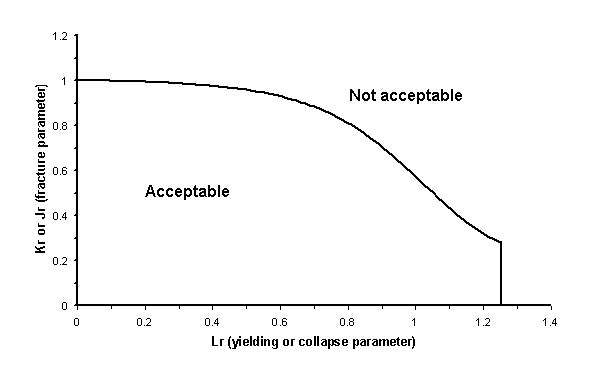Figure 1 A typical failure assessment diagram (FAD).

Figure 1 A typical failure assessment diagram (FAD).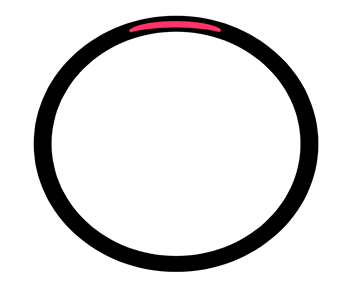Figure 2 Idealised curved elliptical embedded flaw in a pipe (located at 12 o'clock position)

Figure 2 Idealised curved elliptical embedded flaw in a pipe (located at 12 o’clock position)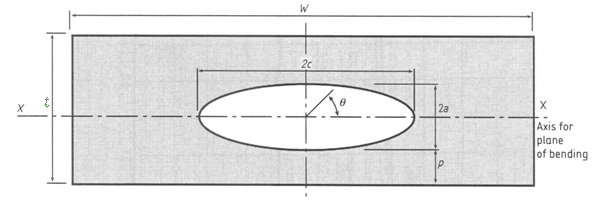Figure 3 Idealised elliptical embedded flaw in a flat plate, used in BS 7910 (BSI, 2005).

Figure 3 Idealised elliptical embedded flaw in a flat plate, used in BS 7910 (BSI, 2005).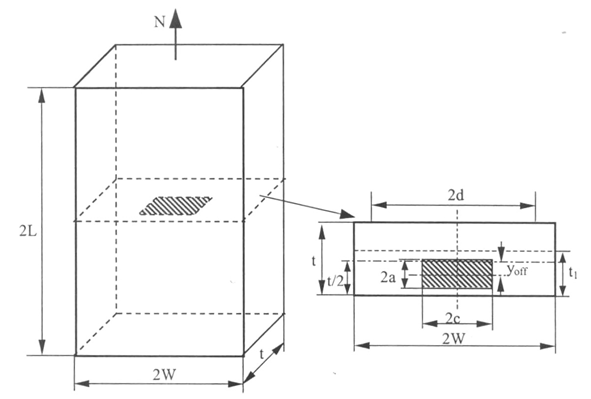Figure 4 Idealised rectangular embedded flaw in a flat plate subjected to tension and/or bending loading, used in R6 (BEGL, 2001).

Figure 4 Idealised rectangular embedded flaw in a flat plate subjected to tension and/or bending loading, used in R6 (BEGL, 2001).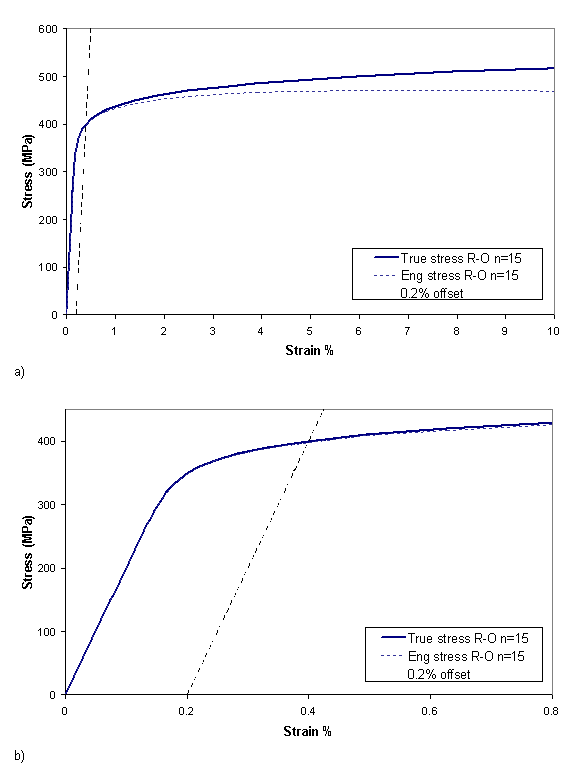Figure 5 Stress strain model used in all but two of the finite element analyses curve up to: a) 10% strain; b) 0.8% strain.

Figure 5 Stress strain model used in all but two of the finite element analyses curve up to:
a) 10% strain;
b) 0.8% strain.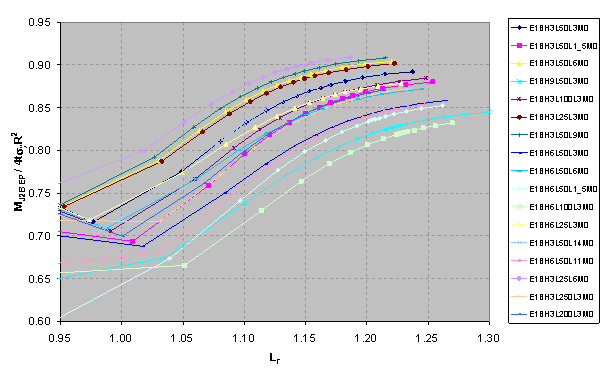Figure 6 MJ2B EP /4tσyRm2 (Je based on elastic-plastic pipe bending stress) vs. Lr.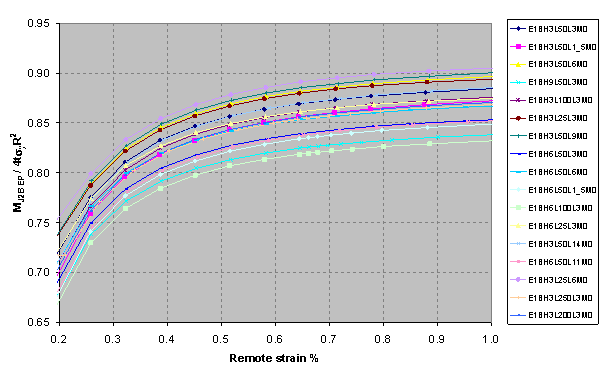Figure 7 MJ2B EP /4tσyRm2 (Je based on elastic-plastic pipe bending stress) vs. remote strain (%).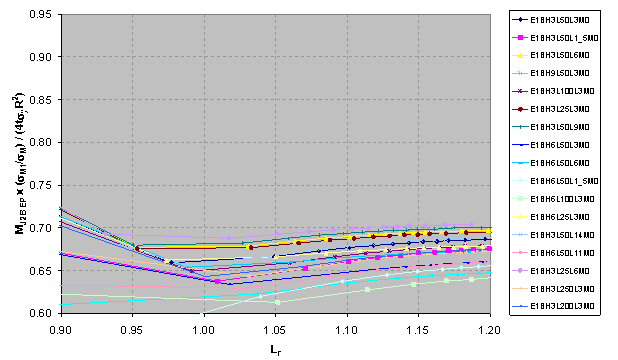Figure 8 MJ2B EP x (σM1/σM) / 4tσyRm2 (Je based on elastic-plastic pipe bending stress) vs. Lr.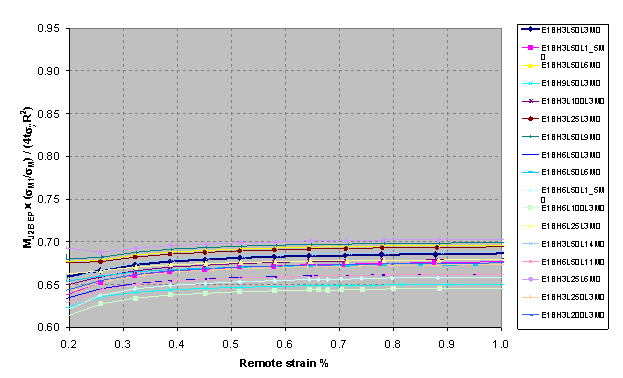Figure 9 MJ2B EP x (σM1/σM) / 4tσyRm2 (Je based on elastic-plastic pipe bending stress) vs. remote strain (%).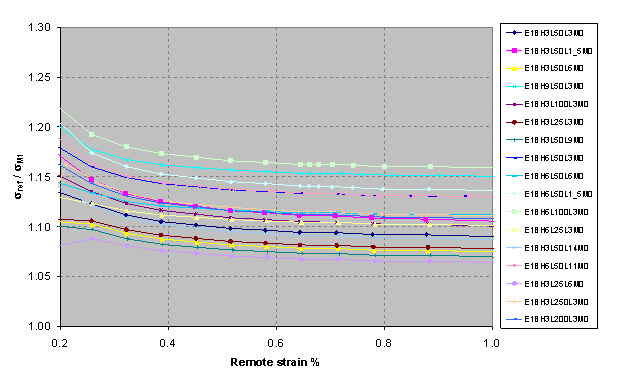Figure 10 Non-dimensional reference stress (σref/σM1) with Je based on elastic-plastic pipe bending stress) vs. remote strain %.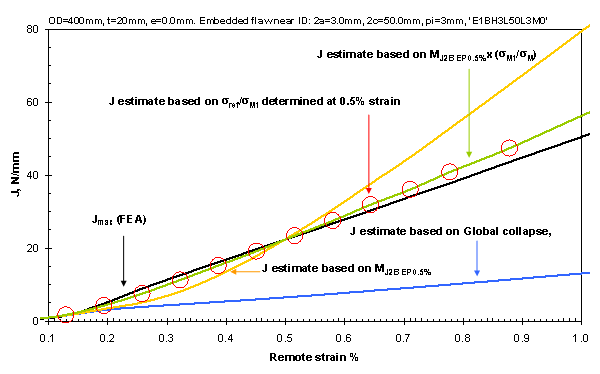Figure 11 J resultsfor model E1BH3L50L3M0 (from FEA and based on estimates of limit moment).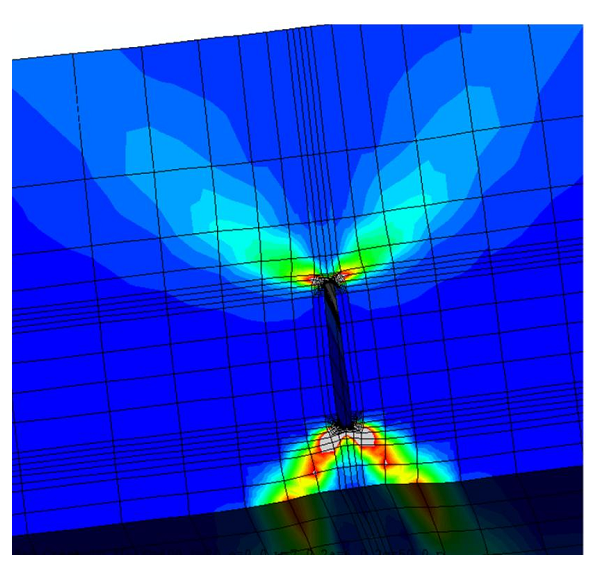Figure 12 Contour plots for the equivalent plastic strain in the ligaments adjacent to an embedded flaw (model E1BH6L50L3M0).